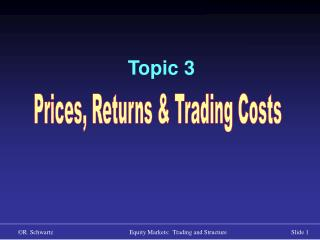DownloadDownload PresentationTopic 3

# Topic 3

Télécharger la présentation## Topic 3

- - - - - - - - - - - - - - - - - - - - - - - - - - - E N D - - - - - - - - - - - - - - - - - - - - - - - - - - -
##### Presentation Transcript

1. Topic 3 Prices, Returns & Trading Costs

2. Returns Measurement

3. Prices(P) P0 , P1 , P2 , … , PT Time P0 P1 P2 PT

4. Arithmetic Returns (P) P0,1 = P1 – P0 P1,2 = P2 – P1 PT-1,T = PT – PT-1

5. Percentage Returns (r) r0,1 = ( P1 – P0 ) / P0 = P0,1 / P0 r1,2 = ( P2 – P1 ) / P1 = P1,2 / P1 rT-1,T = ( PT – PT-1 ) / PT-1 = PT-1,T / PT-1

6. Price Relatives (PR) PR0,1 = P1 / P0 = ( P0 + P0,1 ) / P0 =1 + r0,1 PR1,2 = P2 / P1 = ( P1 + P1,2 ) / P1 = 1 + r1,2 PRT-1,T = PT / PT-1 = ( PT-1 + PT-1,T ) / PT-1 = 1 + rT-1,T PR0,T = PT / P0 = (P1 / P0) * (P2 / P1) *…* (PT / PT-1)

7. Log Returns (R) R = ln(1+r) = ln(price relative) R0,1 = ln(P1/P0)= ln(P1) – ln(P0) = ln(1+r0,1) R1,2 = ln(P2/P1) = ln(P2) – ln(P1) = ln(1+r1,2) RT-1,T = ln(PT) – ln(PT-1) = ln(1+rT-1,T) PR0,T = PT / P0 = (P1 / P0) * (P2 / P1) *…* (PT / PT-1) R0,T = R0,1 + R1,2 +…+ RT-1,T = Ri-1,i = ln(PT) – ln(P0)

8. Question: Which of the following may be normally distributed? P r PR R

9. Two Period Log Returns P2 = P0 ( 1 + r0,2 ) P2 = P0 ( 1 + r0,1 ) ( 1 + r1,2 ) 1 + r0,2 = P2 / P0 = ( P1 / P0 ) * ( P2 / P1 ) = = ( 1 + r0,1 ) ( 1 + r1,2 ) R0,2 = R0,1 + R1,2

10. P* Returns in TraderEx • When P* follows a random walk, P* returns are generated by draws from two distributions: • Poisson distribution (when does P* jump) • A lognormal distribution (how big is the jump) • Ln(P*t ) = Ln(P*t-1 ) + Rt the jump

11. Means and Variances

12. Log Returns: Two Period Mean Assume a constant Mean: E(R0,1) = E(R1,2) E(R0,2) = E(R0,1) + E(R1,2) E(R0,2) = 2E(R0,1)

13. Log Returns: Two Period Variance Var(R0,2)=Var(R0,1)+Var(R1,2)+2Cov(R0,1,R1,2) Assume a constant Variance: Var(R0,1) = Var(R1,2) For Cov(R0,1,R1,2) = 0 Var (R0,2) = 2 Var(R0,1) What if Cov(R0,1,R1,2) < 0 ?

14. Costs

15. Trading Costs 1.Explicit costs • commissions • taxes • etc. 2.Execution Costs(the implicit costs of trading) • Bid-ask spread • Market impact • Delay/opportunity cost • Implementation shortfall

16. From Trading Costs to Volatility • The bid-ask spread • Market impact • Momentum trading • Imperfect price discovery Trading costs cause prices to bounce between higher and lower values

17. Price Time Trading Costs & Volatility C = Implicit transaction cost of buy or sell = Transaction price (triggered by buy order) = Transaction price (triggered by sell order) = Magnitude of C = Unobserved, costless trading price P* P*

18. Trading Costs & Volatility C = Implicit transaction cost of buy or sell = Observed price of buy-triggered trade = Observed price of sell-triggered trade = C = Unobserved, costless trading price P* Price P* Time

19. Trading Costs & Returns Price P T Time

20. Which is More Volatile? P* or the transaction price that we observe? Price P* Observed Transaction Price Time